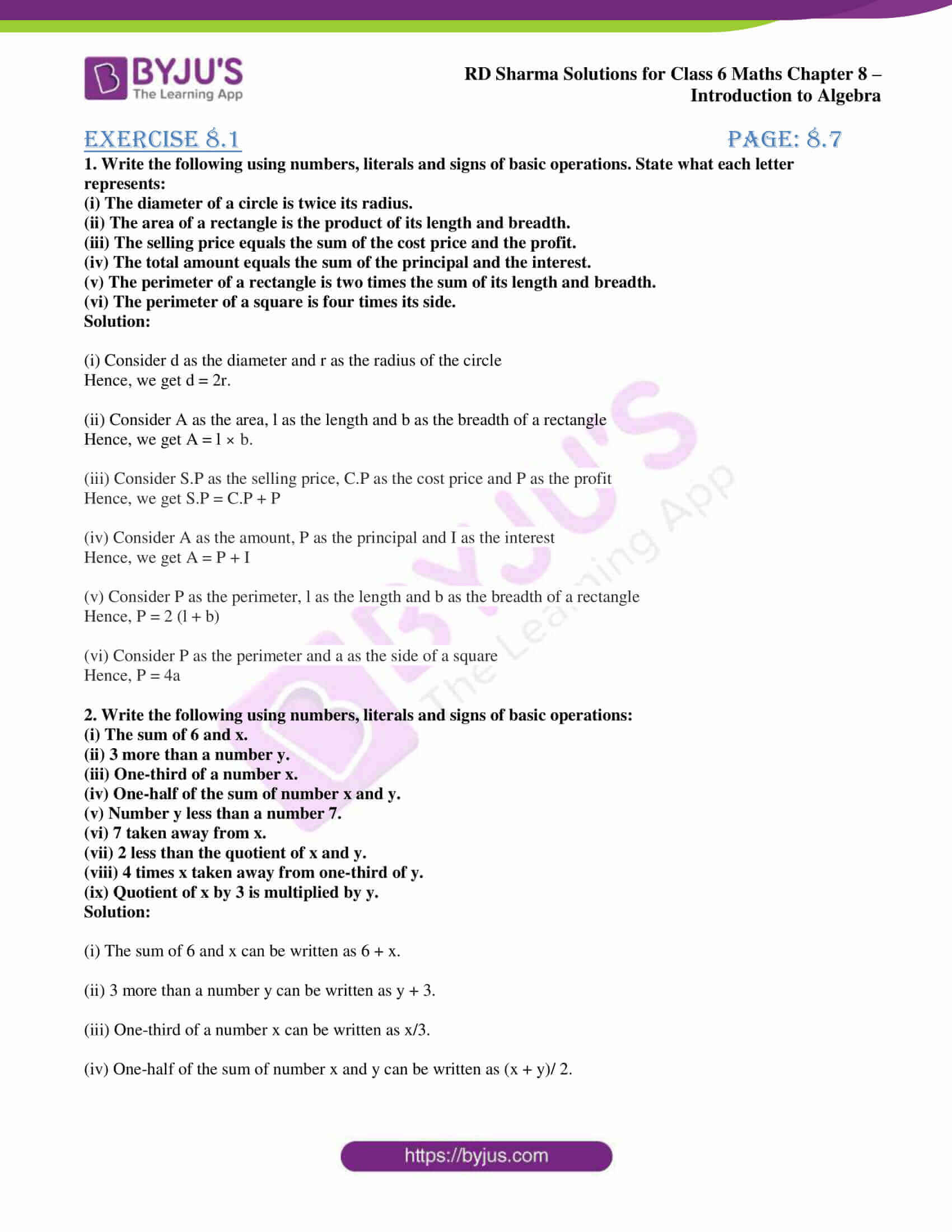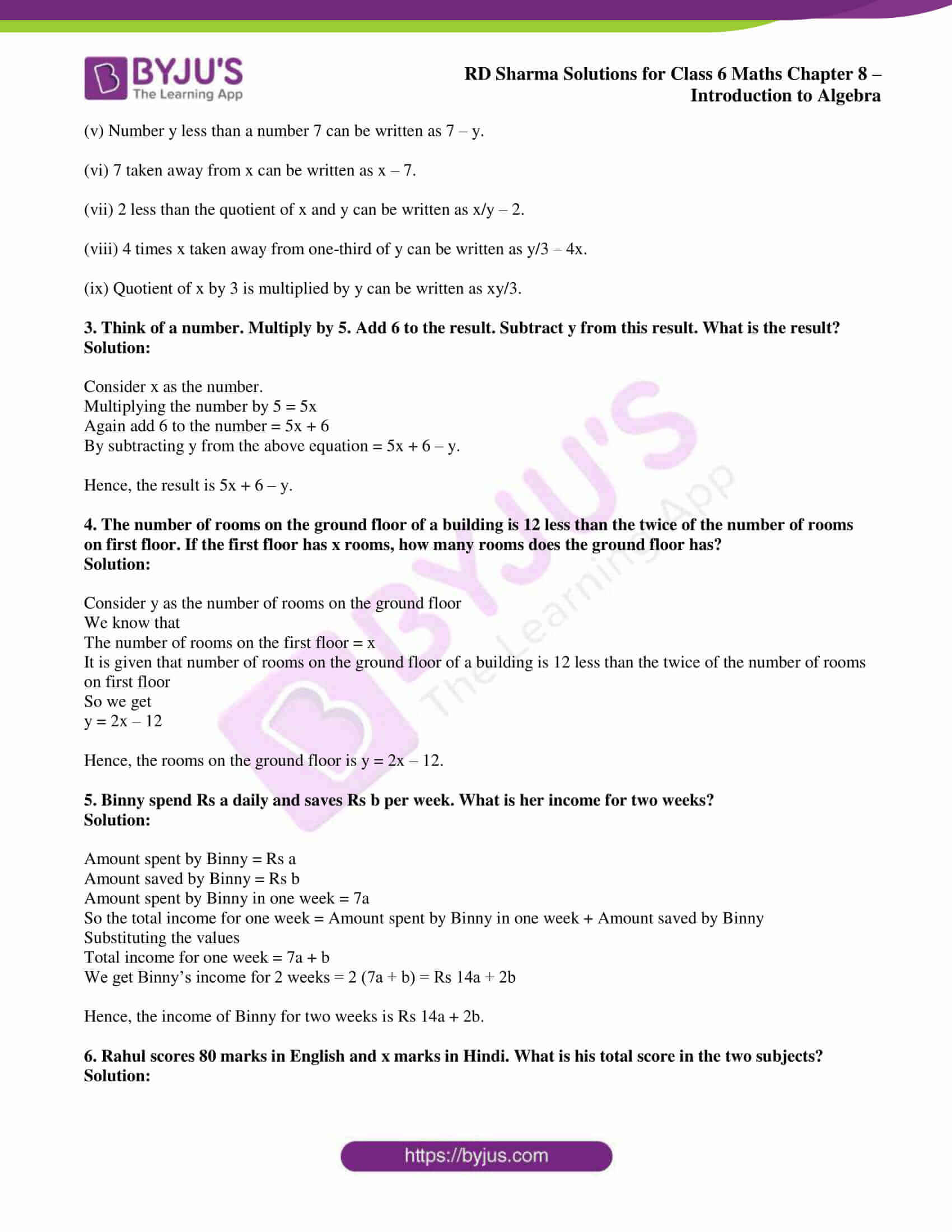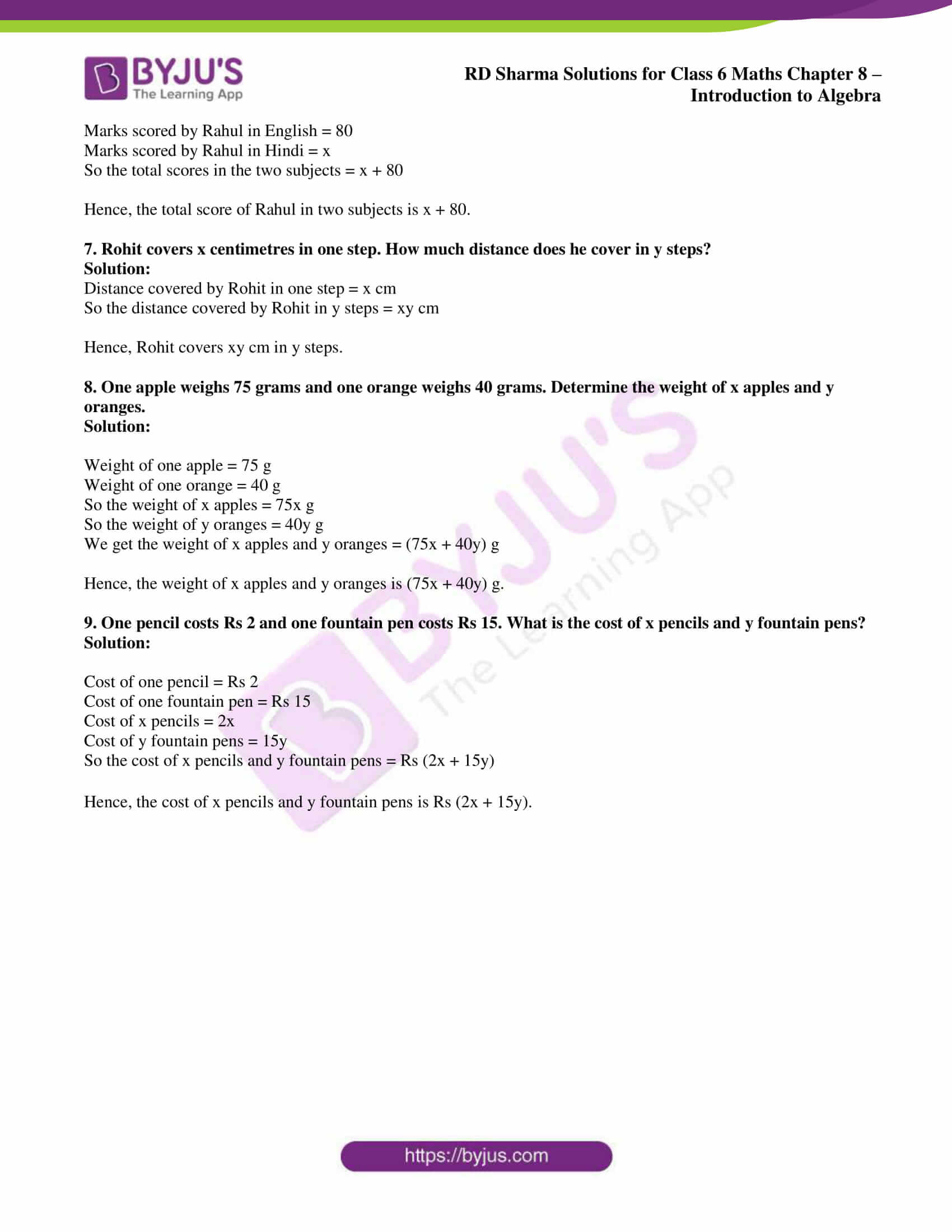# RD Sharma Solutions for Class 6 Maths Chapter 8: Introduction to Algebra Exercise 8.1

Exercise 8.1 consists of topics like the use of letters to denote numbers and basic operations like addition, subtraction, multiplication and division on literals and numbers. RD Sharma Solutions Class 6 are prepared by teachers at BYJU’S having vast experience in the education industry. To know more about the concepts explained in this exercise students can download RD Sharma Solutions for Class 6 Maths Chapter 8 Introduction to Algebra Exercise 8.1 PDF which are given below.

## RD Sharma Solutions for Class 6 Maths Chapter 8: Introduction to Algebra Exercise 8.1 Download PDF### Access answers to Maths RD Sharma Solutions for Class 6 Chapter 8: Introduction to Algebra Exercise 8.1

1. Write the following using numbers, literals and signs of basic operations. State what each letter represents:

(i) The diameter of a circle is twice its radius.

(ii) The area of a rectangle is the product of its length and breadth.

(iii) The selling price equals the sum of the cost price and the profit.

(iv) The total amount equals the sum of the principal and the interest.

(v) The perimeter of a rectangle is two times the sum of its length and breadth.

(vi) The perimeter of a square is four times its side.

Solution:

(i) Consider d as the diameter and r as the radius of the circle

Hence, we get d = 2r.

(ii) Consider A as the area, l as the length and b as the breadth of a rectangle

Hence, we get A = l × b.

(iii) Consider S.P as the selling price, C.P as the cost price and P as the profit

Hence, we get S.P = C.P + P

(iv) Consider A as the amount, P as the principal and I as the interest

Hence, we get A = P + I

(v) Consider P as the perimeter, l as the length and b as the breadth of a rectangle

Hence, P = 2 (l + b)

(vi) Consider P as the perimeter and a as the side of a square

Hence, P = 4a

2. Write the following using numbers, literals and signs of basic operations:

(i) The sum of 6 and x.

(ii) 3 more than a number y.

(iii) One-third of a number x.

(iv) One-half of the sum of number x and y.

(v) Number y less than a number 7.

(vi) 7 taken away from x.

(vii) 2 less than the quotient of x and y.

(viii) 4 times x taken away from one-third of y.

(ix) Quotient of x by 3 is multiplied by y.

Solution:

(i) The sum of 6 and x can be written as 6 + x.

(ii) 3 more than a number y can be written as y + 3.

(iii) One-third of a number x can be written as x/3.

(iv) One-half of the sum of number x and y can be written as (x + y)/ 2.

(v) Number y less than a number 7 can be written as 7 – y.

(vi) 7 taken away from x can be written as x – 7.

(vii) 2 less than the quotient of x and y can be written as x/y – 2.

(viii) 4 times x taken away from one-third of y can be written as y/3 – 4x.

(ix) Quotient of x by 3 is multiplied by y can be written as xy/3.

3. Think of a number. Multiply by 5. Add 6 to the result. Subtract y from this result. What is the result?

Solution:

Consider x as the number.

Multiplying the number by 5 = 5x

Again add 6 to the number = 5x + 6

By subtracting y from the above equation = 5x + 6 – y.

Hence, the result is 5x + 6 – y.

4. The number of rooms on the ground floor of a building is 12 less than the twice of the number of rooms on first floor. If the first floor has x rooms, how many rooms does the ground floor has?

Solution:

Consider y as the number of rooms on the ground floor

We know that

The number of rooms on the first floor = x

It is given that number of rooms on the ground floor of a building is 12 less than the twice of the number of rooms on first floor

So we get

y = 2x – 12

Hence, the rooms on the ground floor is y = 2x – 12.

5. Binny spend Rs a daily and saves Rs b per week. What is her income for two weeks?

Solution:

Amount spent by Binny = Rs a

Amount saved by Binny = Rs b

Amount spent by Binny in one week = 7a

So the total income for one week = Amount spent by Binny in one week + Amount saved by Binny

Substituting the values

Total income for one week = 7a + b

We get Binny’s income for 2 weeks = 2 (7a + b) = Rs 14a + 2b

Hence, the income of Binny for two weeks is Rs 14a + 2b.

6. Rahul scores 80 marks in English and x marks in Hindi. What is his total score in the two subjects?

Solution:

Marks scored by Rahul in English = 80

Marks scored by Rahul in Hindi = x

So the total scores in the two subjects = x + 80

Hence, the total score of Rahul in two subjects is x + 80.

7. Rohit covers x centimetres in one step. How much distance does he cover in y steps?

Solution:

Distance covered by Rohit in one step = x cm

So the distance covered by Rohit in y steps = xy cm

Hence, Rohit covers xy cm in y steps.

8. One apple weighs 75 grams and one orange weighs 40 grams. Determine the weight of x apples and y oranges.

Solution:

Weight of one apple = 75 g

Weight of one orange = 40 g

So the weight of x apples = 75x g

So the weight of y oranges = 40y g

We get the weight of x apples and y oranges = (75x + 40y) g

Hence, the weight of x apples and y oranges is (75x + 40y) g.

9. One pencil costs Rs 2 and one fountain pen costs Rs 15. What is the cost of x pencils and y fountain pens?

Solution:

Cost of one pencil = Rs 2

Cost of one fountain pen = Rs 15

Cost of x pencils = 2x

Cost of y fountain pens = 15y

So the cost of x pencils and y fountain pens = Rs (2x + 15y)

Hence, the cost of x pencils and y fountain pens is Rs (2x + 15y).